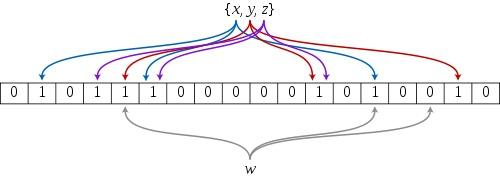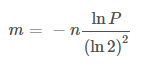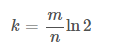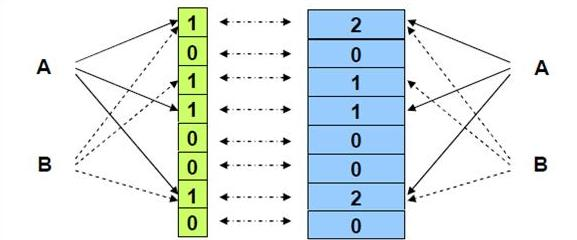# 浅谈布隆过滤器Bloom Filter

### Bloom Filter简介• 存在误判。布隆过滤器可以100%确定一个元素不在集合之中，但不能100%确定一个元素在集合之中。当k个位都为1时，也有可能是其它的元素将这些bit置为1的。
• 删除困难。一个放入容器的元素映射到位图的k个位置上是1，删除的时候不能简单的直接全部置为0，可能会影响其他元素的判断。

### Bloom Filter实现Python中已经有实现布隆过滤器的包：pybloom

pip install pybloom


class BloomFilter(object):
FILE_FMT = b'<dQQQQ'

def __init__(self, capacity, error_rate=0.001):
"""Implements a space-efficient probabilistic data structure
capacity
this BloomFilter must be able to store at least *capacity* elements
while maintaining no more than *error_rate* chance of false
positives
error_rate
the error_rate of the filter returning false positives. This
determines the filters capacity. Inserting more than capacity
elements greatly increases the chance of false positives.
>>> b = BloomFilter(capacity=100000, error_rate=0.001)
False
>>> "test" in b
True
"""
if not (0 < error_rate < 1):
raise ValueError("Error_Rate must be between 0 and 1.")
if not capacity > 0:
raise ValueError("Capacity must be > 0")
# given M = num_bits, k = num_slices, P = error_rate, n = capacity
#       k = log2(1/P)
# solving for m = bits_per_slice
# n ~= M * ((ln(2) ** 2) / abs(ln(P)))
# n ~= (k * m) * ((ln(2) ** 2) / abs(ln(P)))
# m ~= n * abs(ln(P)) / (k * (ln(2) ** 2))
num_slices = int(math.ceil(math.log(1.0 / error_rate, 2)))
bits_per_slice = int(math.ceil(
(capacity * abs(math.log(error_rate))) /
(num_slices * (math.log(2) ** 2))))
self._setup(error_rate, num_slices, bits_per_slice, capacity, 0)
self.bitarray = bitarray.bitarray(self.num_bits, endian='little')
self.bitarray.setall(False)

def _setup(self, error_rate, num_slices, bits_per_slice, capacity, count):
self.error_rate = error_rate
self.num_slices = num_slices
self.bits_per_slice = bits_per_slice
self.capacity = capacity
self.num_bits = num_slices * bits_per_slice
self.count = count
self.make_hashes = make_hashfuncs(self.num_slices, self.bits_per_slice)

def __contains__(self, key):
"""Tests a key's membership in this bloom filter.
>>> b = BloomFilter(capacity=100)
False
>>> "hello" in b
True
"""
bits_per_slice = self.bits_per_slice
bitarray = self.bitarray
hashes = self.make_hashes(key)
offset = 0
for k in hashes:
if not bitarray[offset + k]:
return False
offset += bits_per_slice
return True

        for k in hashes:
if not skip_check and found_all_bits and not bitarray[offset + k]:
found_all_bits = False
self.bitarray[offset + k] = True
offset += bits_per_slice

>>> import math
>>> abs(math.log(0.001))/(math.log(2)**2)
14.37758756605116

>>> import math
>>> abs(math.log(0.05))/(math.log(2)**2)
6.235224229572683

>>> math.e**-((320/50.0)*(math.log(2)**2))
0.04619428041606246

### 应用

1. 缓存穿透

2. 爬虫

3. 垃圾邮件地址过滤

5. Summary Cache

Summary Cache是一种用于代理服务器Proxy之间共享Cache的协议。可以使用布隆过滤器构建Summary Cache，每一个Cache的网页由URL唯一标识，因此Proxy的Cache内容可以表示为一个URL列表。进而我们可以将URL列表这个集合用布隆过滤器表示。

### 扩展posted @ 2019-08-03 17:11  再见紫罗兰  阅读(1596)  评论(0编辑  收藏  举报